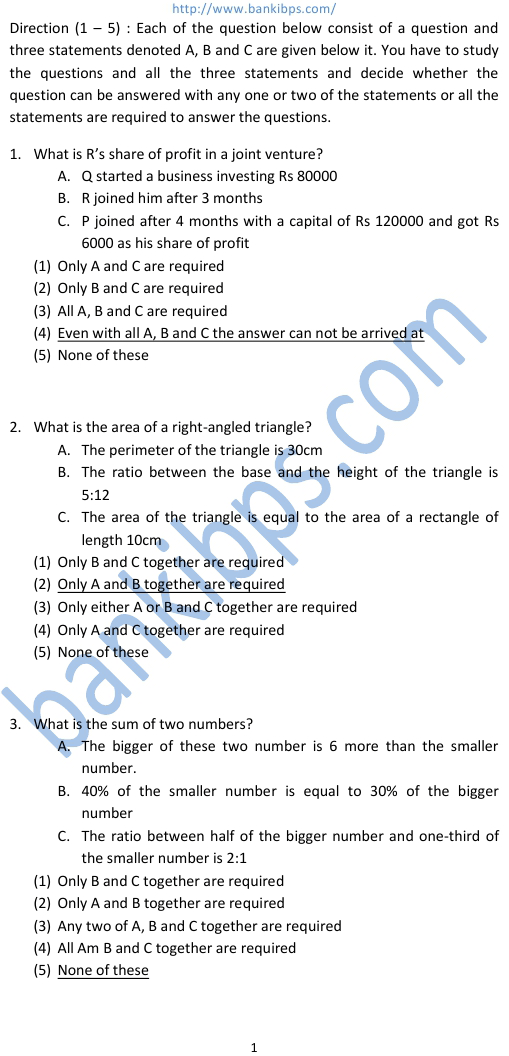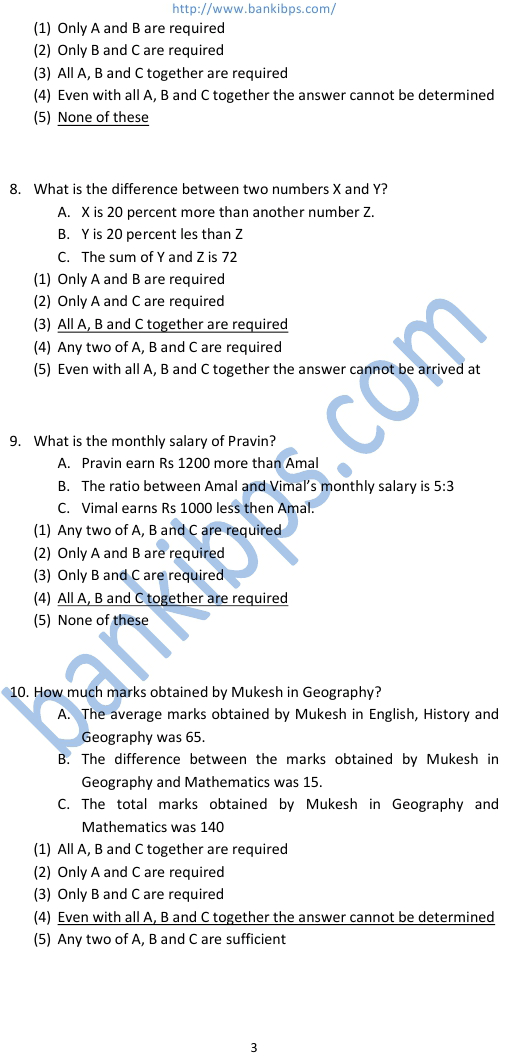# Data Sufficiency

Direction (1 – 5) : Each of the question below consist of a question and three statements denoted A, B and C are given below it. You have to study the questions and all the three statements and decide whether the question can be answered with any one or two of the statements or all the statements are required to answer the questions. 1. What is R’s share of profit in a joint venture? data sufficiency A. Q started a business investing Rs 80000 B. R joined him after 3 months C. P joined after 4 months with a capital of Rs 120000 and got Rs 6000 as his share of profit (1) Only A and C are required (2) Only B and C are required (3) All A, B and C are required (4) Even with all A, B and C the answer can not be arrived at (5) None of these 2. What is the area of a right-angled triangle? A. The perimeter of the triangle is 30cm B. The ratio between the base and the height of the triangle is 5:12 C. The area of the triangle is equal to the area of a rectangle of length 10cm (1) Only B and C together are required (2) Only A and B together are required (3) Only either A or B and C together are required (4) Only A and C together are required (5) None of these 3. What is the sum of two numbers? A. The bigger of these two number is 6 more than the smaller number. B. 40% of the smaller number is equal to 30% of the bigger number C. The ratio between half of the bigger number and one-third of the smaller number is 2:1 (1) Only B and C together are required (2) Only A and B together are required (3) Any two of A, B and C together are required (4) All Am B and C together are required (5) None of these
Practice Exercise - 324 [ Data Sufficiency Question Answer ]